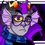# Promotion of a very excellent website

Recently, Cody Johnson created NoBash.com, which is a site dedicated to telling you if you are correct in wanting to give on the problem you are working on and start bashing numbers. Since this arose out of a conversation we were having about a name to give to a math web site, I thought it would be fitting to promote his site.

Ironically, of course. Because I have created a very bashy way to test for primality! See if you can come up with a bashier (slower) primality test than this.

  1 2 3 4 5 6 7 8 9 10 11 12 13 14 15 16 17 18 import time start=time.time() factors=0 for i in range (1,n+1): for j in range (1,i+1): if i%j==0: factors=factors+1 if factors==2: print i, "is prime." if factors!=2: print i, "is not prime." factors=0 end=time.time() print "This operation took", end-start, "seconds."Note by Trevor B.
6 years, 11 months ago

This discussion board is a place to discuss our Daily Challenges and the math and science related to those challenges. Explanations are more than just a solution — they should explain the steps and thinking strategies that you used to obtain the solution. Comments should further the discussion of math and science.

When posting on Brilliant:

• Use the emojis to react to an explanation, whether you're congratulating a job well done , or just really confused .
• Ask specific questions about the challenge or the steps in somebody's explanation. Well-posed questions can add a lot to the discussion, but posting "I don't understand!" doesn't help anyone.
• Try to contribute something new to the discussion, whether it is an extension, generalization or other idea related to the challenge.

MarkdownAppears as
*italics* or _italics_ italics
**bold** or __bold__ bold
- bulleted- list
• bulleted
• list
1. numbered2. list
1. numbered
2. list
Note: you must add a full line of space before and after lists for them to show up correctly
paragraph 1paragraph 2

paragraph 1

paragraph 2

[example link](https://brilliant.org)example link
> This is a quote
This is a quote
    # I indented these lines
# 4 spaces, and now they show
# up as a code block.

print "hello world"
# I indented these lines
# 4 spaces, and now they show
# up as a code block.

print "hello world"
MathAppears as
Remember to wrap math in $$ ... $$ or $ ... $ to ensure proper formatting.
2 \times 3 $2 \times 3$
2^{34} $2^{34}$
a_{i-1} $a_{i-1}$
\frac{2}{3} $\frac{2}{3}$
\sqrt{2} $\sqrt{2}$
\sum_{i=1}^3 $\sum_{i=1}^3$
\sin \theta $\sin \theta$
\boxed{123} $\boxed{123}$

Sort by:

Divide the number by six and check the remainder.exception(2,3).

- 6 years, 11 months ago

Remainder has to 1 or 5 for a number to prime.

- 6 years, 11 months ago

$p\equiv \pm 1\pmod 6$ ($p\in\mathbb P$, $p>3$) is a necessary condition, but not sufficient (e.g., try $25$).

- 6 years, 11 months ago

Right.

- 6 years, 11 months ago

First, create a code that prime factorizes a number. Then, add the number $2$ to your prime array.

Now every step, you take all the primes in your prime array, multiply them, then add one. Then you prime factorize, and if there are any primes in your prime factorization (which there will be) that are not in the array, add them to the array. Repeat. Guaranteed non-efficiency.

I'm not sure that gives all the prime numbers though.

- 6 years, 11 months ago